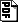Computing Procedure Summaries for Interprocedural Analysis

### Abstract

We describe a new technique for computing procedure summaries for performing an interprocedural analysis on programs. Procedure summaries are computed by performing a backward analysis of procedures, but there are two key new features: (i) information is propagated using ``generic'' assertions (rather than regular assertions that are used in intraprocedural analysis); and (ii) unification is used to simplify these generic assertions (thus generalizing our recent technique of using unification to simplify regular assertions in intraprocedural analysis~\cite{GT:AssertionChecking}).

We describe conditions under which this technique yields efficient interprocedural analyses. We illustrate this technique by applying it to two abstractions: unary uninterpreted functions and linear arithmetic. In the first case, we get a PTIME algorithm for a special case of the long-standing open problem of interprocedural global value numbering (the special case being that we consider unary uninterpreted functions instead of binary). This also requires developing efficient algorithms for manipulating singleton context-free grammars, and builds on an earlier work by Plandowski~\cite{Plandowski94:ESA}. In linear arithmetic case, we get new algorithms for precise interprocedural analysis of linear arithmetic programs with complexity matching that of the best known deterministic algorithm~\cite{Seidl04}.

Long version of the paper:pdf.

#### Slides

In powerpoint: pdf

#### BibTeX Entry

```
@inproceedings{GulwaniTiwari07:ESOP,
author = "S. Gulwani and A. Tiwari",
title = "Computing Procedure Summaries for Interprocedural Analysis",
booktitle = {European Symp. on Programming, ESOP 2007},
year = 2007,
editor = "De Nicola, R.",
series = {LNCS},
volume = 4421,
pages = "253--267",
}

```